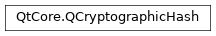# QCryptographicHash¶

The `QCryptographicHash` class provides a way to generate cryptographic hashes. More## Detailed Description¶

`QCryptographicHash` can be used to generate cryptographic hashes of binary or text data.

Refer to the documentation of the `Algorithm` enum for a list of the supported algorithms.

class PySide2.QtCore.QCryptographicHash(method)
param method:

`Algorithm`

Constructs an object that can be used to create a cryptographic hash from data using `method` .

PySide2.QtCore.QCryptographicHash.Algorithm

Note

In Qt versions before 5.9, when asked to generate a SHA3 hash sum, `QCryptographicHash` actually calculated Keccak. If you need compatibility with SHA-3 hashes produced by those versions of Qt, use the `Keccak_` enumerators. Alternatively, if source compatibility is required, define the macro `QT_SHA3_KECCAK_COMPAT` .

Constant

Description

QCryptographicHash.Md4

Generate an MD4 hash sum

QCryptographicHash.Md5

Generate an MD5 hash sum

QCryptographicHash.Sha1

Generate an SHA-1 hash sum

QCryptographicHash.Sha224

Generate an SHA-224 hash sum (SHA-2). Introduced in Qt 5.0

QCryptographicHash.Sha256

Generate an SHA-256 hash sum (SHA-2). Introduced in Qt 5.0

QCryptographicHash.Sha384

Generate an SHA-384 hash sum (SHA-2). Introduced in Qt 5.0

QCryptographicHash.Sha512

Generate an SHA-512 hash sum (SHA-2). Introduced in Qt 5.0

QCryptographicHash.Sha3_224

Generate an SHA3-224 hash sum. Introduced in Qt 5.1

QCryptographicHash.Sha3_256

Generate an SHA3-256 hash sum. Introduced in Qt 5.1

QCryptographicHash.Sha3_384

Generate an SHA3-384 hash sum. Introduced in Qt 5.1

QCryptographicHash.Sha3_512

Generate an SHA3-512 hash sum. Introduced in Qt 5.1

QCryptographicHash.Keccak_224

Generate a Keccak-224 hash sum. Introduced in Qt 5.9.2

QCryptographicHash.Keccak_256

Generate a Keccak-256 hash sum. Introduced in Qt 5.9.2

QCryptographicHash.Keccak_384

Generate a Keccak-384 hash sum. Introduced in Qt 5.9.2

QCryptographicHash.Keccak_512

Generate a Keccak-512 hash sum. Introduced in Qt 5.9.2

Parameters:
Return type:

bool

Reads the data from the open `QIODevice` `device` until it ends and hashes it. Returns `true` if reading was successful.

Parameters:
Parameters:

data – str

Adds the first `length` chars of `data` to the cryptographic hash.

static PySide2.QtCore.QCryptographicHash.hash(data, method)
Parameters:
Return type:

`PySide2.QtCore.QByteArray`

Returns the hash of `data` using `method` .

static PySide2.QtCore.QCryptographicHash.hashLength(method)
Parameters:

method`Algorithm`

Return type:

int

Returns the size of the output of the selected hash `method` in bytes.

PySide2.QtCore.QCryptographicHash.reset()

Resets the object.

PySide2.QtCore.QCryptographicHash.result()
Return type:

`PySide2.QtCore.QByteArray`

Returns the final hash value.